Next: Conclusions Up: Partial inverse heuristic for Previous: Remove linear term heuristic.

# Inverting functions

To generate iterators we often need to invert functions or expressions. For example, if at one point of our isolation process we have the equation

x2 = h(x)

we derive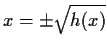. The choice of signs presents a problem. The easiest way to resolve it is to derive two iterators, one for each sign as we have done before. Almost all functions except for lineal polynomials, present this or other problems for isolation.

An alternative to computing inverses which are multiple-valued is the following. We want to compute a value xi+1 from a previous value xi. When there are multiple choices for xi+1we want to select the one with minimal distance to xi for convergence reasons. I.e. select the xi+1 which makes | xi+1-xi| minimal. This observation is a crucial one for our algorithm. So from xi+12 = h(xi) we will select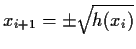, the sign depending on whether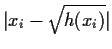or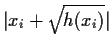is smaller. This resolves the first problem, the choice between multiple values. For isolating, we use a function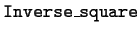. E.g. from g(xi+1)2 = h(xi) we derive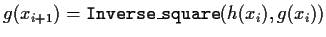, where

  Inverse_square := proc( rhs, lhs )
r := sqrt( rhs );
if abs(r-lhs) <= abs(r+lhs) then r else -r fi
end;

In summary, when the result of an isolating step is a multivalued function, we can take two approaches
(a)
Enumerate all iterators, either explicitly as in the case for x2 = h(x) or as a function of an arbitrary integer n as for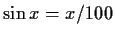.
(b)
Leave the choice to be determined during the actual computation, by choosing the one closest to the previous value, and hence improving the chances of convergence.

A second problem that we have with inverting functions is a domain problem, and it can be illustrated with the equation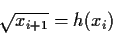from which we isolate xi+1 = h(xi)2. In this case there is no choice of inverse, but if h(xi) is negative (or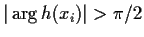) then the inverse cannot be computed, as we assume thatreturns its principal value. E.g. for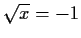, isolation gives x=1 which is not a solution of the original equation. Such a situation should be treated as a domain error. In this case we use a new function to define this type of manipulation,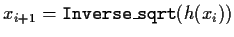and
  Inverse_sqrt := proc( rhs )
a := argument( rhs );
if a <= -Pi/2 or a > Pi/2 then
ERROR( cannot invert sqrt )
else rhs^2 fi
end;Next: Conclusions Up: Partial inverse heuristic for Previous: Remove linear term heuristic.
Gaston Gonnet
1998-07-08